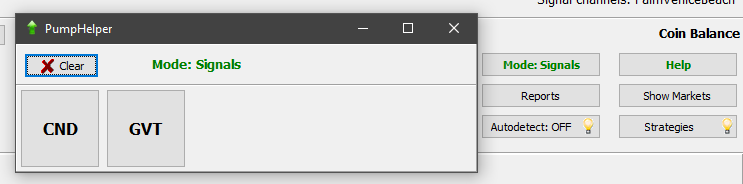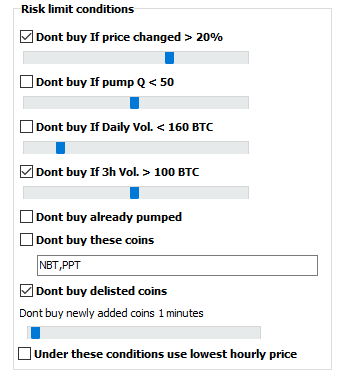How it works: first you should configure the pump detector strategy (settings example at the bottom of this page). Configure it such way that it gives about 3-5 signals per minute. You MUST turn off autobuy in the strategy also you may turn off auto opening charts. Then activate the strategy, turn ON “autodetect”, go to the Menu and select “Pump Tool”. This brings the window with market buttons which the detector has found:Of course there will be false detections, thats why you turned OFF the autobuy option. Now all you have to do is wait the pump channel and as soon as you see the coin, push appropriate button in the Pump Helper. In the “signals” mode this will immediately buy the coin. In the “manual” mode the button only opens the chart. Note: the button is NOT related to the pump detetor strategy settings; its just a button to help you faster buy the coin. The settings it uses for order’s handling are the settings from the main window ! When preparing to a pump pay attention to the following settings: 1. In the main window its strongly recommended  to use “buy for 30-secs old price”, this will use limit order related to initial coin’s price. Otherwise using market price is way too risky!2. Settings -> Main: For well-know pumps you may turn off all checkboxes in the picture below:If you don’t know whether to trust a pump group, use these settings to prevent the bot from entering prepumped coins: “Dont buy If price changed more then 10%..15%”, “If 3h volume more then like 50 BTC” and so on. IMPORTANT: If the bot has not bought the coin (due to risk limit settings or the prise raised up as hell and you missed it)  – cancel your order and relax, or at least move the order down, but NEVER move it up!   The pump detector strategy example: (If you want less sensitivity, adjust the following:) PumpPriceRaise=0.3000 (use higher value like 0.5 .. 1%) PumpBuysPerSec=3 PumpVolPerSec=0.2000 (use higher value like 1-2 BTC for strong pumps) PumpBuyersPerSecMin=1 PumpBuyersPerSecMax=40 (use lower value like 4-10) PumpVolEMA=4.000 (2 instead of 4) ##Begin_Strategy Active=-1 StrategyName=Binance Pump Working Comment= SignalType=PumpsDetection ChannelName= ChannelKey= SilentNoCharts=YES ReportToTelegram=NO SoundAlert=NO SoundKind= KeepAlert=60 CoinsWhiteList= CoinsBlackList=NBT MinVolume=0 MaxVolume=3000 MinHourlyVolume=0 MaxHourlyVolume=150 PenaltyTime=300 Delta_3h_Min=0.0000 Delta_3h_Max=50.00 NextDetectPenalty=30 AutoBuy=NO MaxActiveOrders=1 AutoCancelBuy=25 AutoCancelLowerBuy=60 BuyType=Buy OrderSize=0.0000 buyPrice=4.000 Use30SecOldASK=YES TlgUseBuyDipWords=YES TlgBuyDipPrice=-10.5000 SellPrice=10.000 PriceDownTimer=0 PriceDownDelay=10 PriceDownPercent=0.2000 PriceDownRelative=NO PriceDownAllowedDrop=0.1000 UseSignalStops=YES UseStopLoss=NO StopLossDelay=0 StopLoss=2.000 StopLossSpread=0.8000 AllowedDrop=0.0000 UseSecondStop=NO TimeToSwitch2Stop=120 SecondStopLoss=2.000 UseStopLoss3=NO TimeToSwitchStop3=60 StopLoss3=3.000 AllowedDrop3=1.0000 UseTrailing=NO TrailingPersent=-1.0000 TrailingSpread=0.8000 UseTakeProfit=NO TakeProfit=1.500 DropsMaxTime=600 DropsPriceMA=10 DropsLastPriceMA=1 DropsPriceDelta=2.000 DropsPriceIsLow=NO WallsMaxTime=0 WallsPriceDelta=0.0000 WallBuyVolDeep=0.0000 WallBuyVolume=0 WallBuyVolToDailyVol=0.0000 WallSellVolToBuy=0.0000 WallSellVolDeep=0.0000 PumpPriceRaise=0.3000 PumpBuysPerSec=3 PumpVolPerSec=0.2000 PumpBuyersPerSecMin=1 PumpBuyersPerSecMax=40 PumpVolEMA=4.000 PumpBuyersInterval=4.000 PumpMoveTimer=3 PumpMovePersent=0.5000 MShotPriceMin=2.000 MShotPrice=7.000 MShotAddHourlyDelta=0.1000 MShotSellAtLastPrice=YES MShotSellPriceAdjust=0.0000 MShotReplaceDelay=0.0000 MShotRaiseWait=30.00 MShotSortBy=Last2hDelta VolShortInterval=5 VolShortPriseRaise=0.5000 VolLongInterval=1800 VolBvShortToLong=3.000 VolBvLongToHourlyMin=0.2000 VolBvLongToHourlyMax=2.000 VolBvLongToDailyMin=0.2000 VolBvLongToDailyMax=2.000 VolBvToSvShort=5.000 VolBvShort=0.5000 VolBuyersShort=3 VolSvLong=0.4000 VolTakeLongMaxP=NO VolAtMinP=1.500 VolAtMaxP=0.5000 VolDeltaAtMaxP=0.5000 VolDeltaAtMinP=0.2000 volBidsDeep=3.000 volBids=0.5000 volAsksDeep=4.000 volBidsToAsks=1.500 VLiteT0=300 VLiteT1=180 VLiteT2=180 VLiteT3=180 VLiteP1=1.0000 VLiteP2=1.0000 VLiteP3=1.0000 VLiteMaxP=5.000 VLitePDelta1=0.0000 VLitePDelta2=0.0000 VLiteDelta0=2.000 VLiteMaxSpike=7.000 VLiteV1=1.0000 VLiteV2=1.0000 VLiteV3=1.0000 VLiteWeightedAvg=YES VLiteReducedVolumes=NO WavesT0=300 WavesT1=180 WavesT2=180 WavesT3=180 WavesP1=1.0000 WavesP2=-11.0000 WavesP3=1.0000 WavesDelta0=2.000 WavesMaxSpike=7.000 WavesV1=1.0000 WavesV2=1.0000 WavesV3=1.0000 WavesWeightedAvg=YES WavesReducedVolumes=NO DeltaInterval=600 DeltaShortInterval=5 DeltaPrice=2.000 DeltaVol=5.000 DeltaVolRaise=2.000 DeltaVolSec=0.1000 DeltaBuyers=15 DeltaLastPrice=0.3000 ComboStart= ComboEnd= ComboDelayMin=0 ComboDelayMax=600 ##End_Strategy#